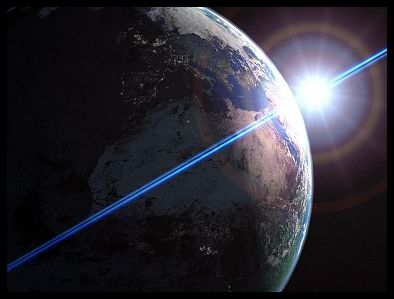Astronomy 161 An Introduction to Solar System Astronomy Prof. Scott Gaudi

# Lecture 19: Orbits

## Key Ideas:

Newton generalized Kepler's laws to apply to any two bodies orbiting each other
• First Law: Orbits are conic sections with the center-of-mass of the two bodies at the focus.
• Second Law: angular momentum conservation.
• Generalized Third Law that depends on the masses of the two bodies.
First Triumph of Newtonian Gravity
• The prediction of the return of Halley's Comet

## Kepler's Laws Revisited

Kepler's Laws of Planetary Motion are as follows:

First Law:
Planets orbit on ellipses with the Sun at one focus.

Second Law:
Planet sweeps out equal areas in equal times.

Third Law:
Period squared is proportional to the size of the semi-major axis cubed.
Expressed Mathematically as: P2=a3, for P in years and a in AUs.

## Newton's Generalization

Newton showed that Kepler's Laws can be derived from

• The Three Laws of Motion
• The Law of Universal Gravitation.

Further, Newton generalized the laws to apply to any two bodies moving under the influence of their mutual gravitation. For example, these laws apply equally to

• The Moon orbiting the Earth.
• A space probe orbiting the Moon.
• Two stars orbiting each other.
and so on...

## First Law of Orbital Motion

The shape of an orbit is a conic section with the center of mass at one focus.
There are two parts to Newton's formulation of Kepler's First Law:

Shapes of Orbits are Conic Sections:

• Curves found by cutting a cone with a plane.
• Circles, Ellipses, Parabolas, and Hyperbolas

The Center of Mass is at the Focus:

• Strictly speaking, it is not just the Earth orbitting the Sun. The Earth and Sun orbit each other about their mutual Center of Mass.
Because the Earth is so much smaller than the Sun, their mutual center of mass is inside the Sun, so the difference is not immediately apparent.

## Conic Section Curves

These are curves formed by the intersection of a cone and a plane cutting it at various angles.

Conic curves come in two families:

Closed Curves:

• Ellipses
• Circles, which are a special case of an ellipse with e=0
• Orbits are bound and objects are trapped in orbit forever around the parent body.

Open Curves:

• Hyperbolas
• Parabolas, which are a special case of a hyperbola
• Orbits are unbound and objects can escape the gravity of the parent body.
Which of these orbits you will be in is determined by your orbital speed. There are two speeds of particular interest...

## Circular Velocity

Velocity needed to sustain a circular orbit of a given radius, r, from a massive body, M: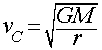• If v<vC, the orbit is an ellipse smaller than the circular orbit.
• Go a little faster than vC, the orbit is an ellipse larger than the circular orbit,
• Go a lot faster, and…

## Escape Velocity

This is the minimum velocity required to have a parabolic orbit starting at a given distance, r, from a massive body, M: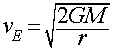At the Earth's surface:

• vC = 7.9 km/sec (28,400 km/hr)
• vE = 11.2 km/sec (40,300 km/hr)
Thus, which orbit you will be in starting from a given point (labeled P here) is determined by your speed at that point:
• If the speed is the circular speed at P (VC above), the orbit will be a circle (red curve).

• If the speed is less than the circular speed at P, the orbit will be an ellipse smaller than the circle (blue curve)

• If the speed is larger than the circular speed at P but less than the escape speed, VE, the orbit will be an ellipse larger than the circle (green curve)

• If the speed is the escape speed from P (VE above), the orbit will open into a Parabola (magenta curve).

• If the speed is greater than the escape speed from P, the orbit will be a Hyperbola (black curve). The greater the speed, the "flatter" (more open) the Hyperbolic curve of the orbit

## Center of Mass

Two objects orbit about their center of mass:

• Balance point between the two masses
• Semi-Major axis: a = a1 + a2
• Relative positions: a2 / a1 = M1 / M2
Example: Earth and Sun
Msun = 2 x 1030 kg
Mearth = 6 x 1024 kg

From the balance relation, the distances of the Sun and Earth from their mutual center of mass are related to the size of the semi-major axis of the Earth's orbit (a) and the ratio of the masses:

asun + aearth = 1 AU = 1.5 x 108 km
asun/aearth = Mearth/Msun = 3 x 10-6

After some simple algebra (do it!), we find:

asun = 450 km

Since the radius of the Sun is 700,000 km, this means that the center-of-mass of the Earth-Sun system is deep inside the Sun.

## Second Law of Orbital Motion

Orbital motions conserve angular momentum.

This doesn't sound much like "equal areas in equal times", but in fact it is the same thing.

Angular Momentum:

L = mvr = constant

Where:
m = mass,
v = velocity,
r = distance from the center of mass.

## Angular Momentum & Equal Areas

Angular Momentum is conserved, which means that L is a constant.

If the distance changes, the velocity must change to compensate so as to keep L constant:

Near Perihelion:

• Planet is closer to the Sun, so r is smaller.
• The speed v must be proportionally faster to compensate.

Near Aphelion:

• Planet is farther to the Sun, so r is larger.
• The speed v must be proportionally slower to compensate.

A familiar example of the same principle at work is a figure skater doing a spin. In an "upright spin", the skater stands on one leg with arms outstretched and spins about an up/down axis. The spin is accelerated by the skater drawing in his/her arms. By drawing in his/her arms, they are moving mass closer to the center of their body, and conservation of angular momentum demands that they spin faster.

## Third Law of Orbital Motion

Newton's Generalization of Kepler's 3rd Law: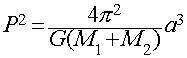Where:
P = period of the orbit
a = semi-major axis of the orbit
M1 = mass of the first body
M2 = mass of the second body

## A Third Law for Every Body

The proportionality between the square of the period and the cube of the semi-major axis now depends on the masses of the two bodies.

For planets orbiting the Sun, Msun is so much bigger than any planet (even Jupiter, at 1/1000th Msun), that we recover Kepler's version of the Third Law from Newton's more general form:Note that the constant of proportionality is the same for all planets (to a good approximation, certainly to within Kepler's data from Tycho).

In Kepler's version, the constant of proportionality works out to be 1.0 if we use units of years for P and AUs for the semi-major axis, a. While computationally convenient, it hides the underlying dependence on the mass of the Sun from sight. The problem with empirical laws, like Kepler's formulation, is that they only show the surface, not the important details underlying them.

## Measuring Masses

Newton's generalized form of Kepler's 3rd law gives us a way to measure masses from orbital motions!

For exampl, we can derive the mass of the Sun by using the period and size of the Earth's orbit:

Pearth = 1 year = 3.156 x 107 seconds
aearth = 1 AU = 1.496 x 1011 meters

Using Newton's Form of Kepler's 3rd law for the solar system above, we see that once we know P and a (G and pi are constants), the only unknown is the Mass of the Sun, which can be solved for easily after a little light algebra: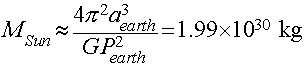(You can verify the numbers for yourself by using G=6.67 x 10-11 Newton m2/kg2, and the values of P and a for the Earth given above in seconds and meters. Do it!).

## A Universal Method for Measuring Masses

The generalized form of Kepler's Third law gives us a powerful tool for measuring the masses of objects by measuring the periods and sizes of their orbits. For example:

We can measure the mass of Jupiter from the orbits of the Galilean moons, since MJupiter>>Mmoons

• Find MJupiter = 300 Mearth

We can measure the total mass of the Earth and Moon system.

• Earth is only ~81x more massive than the Moon, so you have to use the full formula.
• Get the mass of the Earth independently (e.g., our falling bodies experiment from yesterday's lecture).

We can measure the masses of binary stars using the full formula and by observing their orbit parameters (you will see this done in Astronomy 162).

## The Triumph of Newtonian Gravity

Newton's description of planetary positions is only a start.

It also allows quantitative new predictions. An early application was to Halley's Comet:

• Using Newtonian Gravity, Edmund Halley found that the orbit of the great comet of 1682 was similar to comets seen in 1607 and 1537.
• Predicted it would return in 1758/59.
• It did, dramatically confirming Newton's laws.
...

## The Why of Planetary Motions

Kepler's Laws are descriptions of the motion:

• Arrived at by trial and error, and some vague notions about celestial harmonies
• Only describe the motions, without explaining why they move that way.

Newton provides the explanation:

• Kepler's Laws are a natural consequence of Newton's Three Laws of Motion and his law of universal gravitation.
By adding the why, Newton gave his laws predictive power, and allows us to use them as tools to explore the Universe, both figuratively and literally. We can predict new phenomena or understand oddities in the motions (they laws give us a framework in which to interpret data), and we can literally use them to fly spacecraft through the solar system.
Return to [ Unit 4 Index | Astronomy 161 Main Page ]